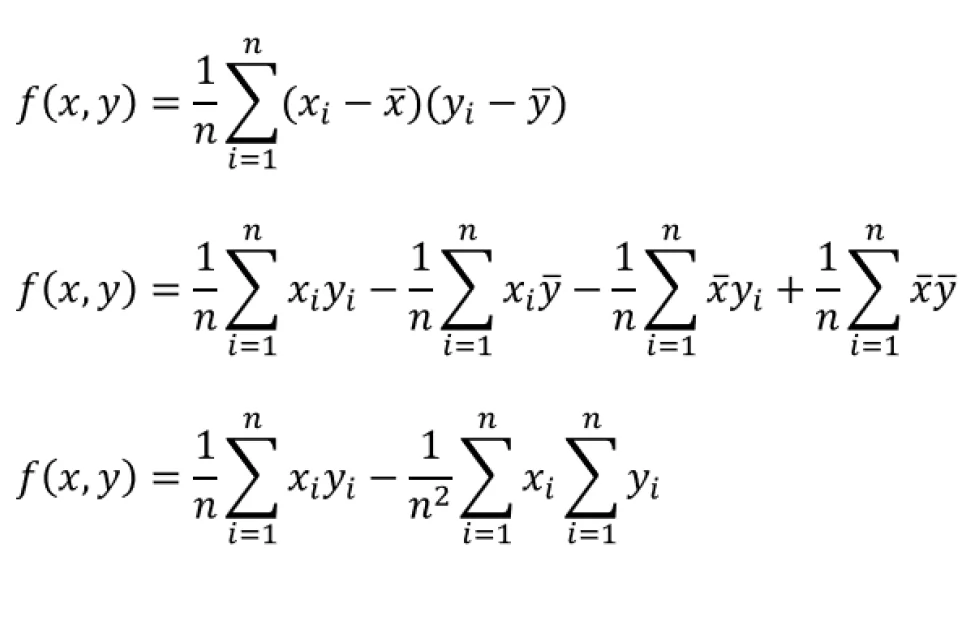Covariance vs. Correlation: Understanding Two Different Concepts Concerning Data Science - Unite.AI

# Covariance vs. Correlation: Understanding Two Different Concepts Concerning Data ScienceUpdated onData science has many interchangeable terms. It is the science of analyzing and understanding data to provide a better solution to an existing problem. It can give accurate predictions of future trends and actions, making it the most popular and trending field of today’s world. Data science uses a combination of algorithms, artificial intelligence, and statistics to understand data behavior. Understanding data to predict future outcomes is the primary target of data science. All the algorithms and machine learning programs are based on statistical relations. Statistics can be considered as the base of data science.

## Statistics

Statistics is a branch of mathematics that deals with data analysis. Standard definitions and techniques are used in statistics to understand and analyze the data behavior. These techniques in the advanced stage become the blocks for machine learning algorithms. The most common and frequently used concept in statistics is variance. Variance is the variation of each entry in the data set from the mean of the data set. Variance defines the divergence and widescreens of the data set concerning its mean or average. Variance is used widely to measure the abnormalities in the data.Covariance and correlation are used interchangeably in statistics. We come across these two terms frequently in statistics. In this field, where people talk about the relationship between two different sets of data, the terms covariance and correlation have a symbiotic relationship. Covariance defines the variation between two variables, while the correlation defines the relationship between two independent variables. Data science uses both concepts regularly. Covariance is used to understand the change in two independent factors in a scenario concerning each other. Correlation talks about the rate of change concerning each other.

## Covariance:

Covariance defines the direction of the relation between two variables. It does not ponder over the strength of the relationship. It lets us know the proportionality between the two variables. Covariance can be any real number. It is dependent on the variance of the variables and the scale of the mapping. It can be calculated as the product of summation of differences of average from the variable set divided by the total number of elements. Covariance in data science is used to analyze the data to understand the past happenings. The behavior of various variables changes with a change in a factor. That can be used to better understand what is happening. Covariance can provide a basic understanding of the relationship between the variables. The variable can either be directly proportional or inversely proportional. The non-proportional variables need other advanced statistical techniques to understand, observe, and study.

## Correlation:

Correlation explains the strength of the relationship between two variables. Covariance and correlation are related. If you divide covariance by the product of the standard deviations of both variables, you get the correlation. Correlation is bound to the set [-1,1]. It enables us to predict one variable depending upon the other one. This is how data science accurately predicts future occurrences. It is an improvised version of covariance. It shows both the relationship between variables and the strength of the variables. Correlation coefficients are used in machine learning to create linear regressions. If the variables are closely related, the coefficient value will be closer to either 1 or -1.

IF the variables are not related linearly, the coefficient will tend to be zero. It does not mean the coefficients are entirely unrelated. They may have a higher-order relationship. The accuracy of a prediction data science model will depend on the coefficient factor. The closer the factor is to the extremes, the more accurately the prediction model’s algorithm works.## Covariance vs. Correlation

The significance and importance of covariance and correlation are very rigidly proved in the current algorithms and usage. Data science relies heavily upon both these linear techniques to analyze and understand big data. Both are very closely related to each other but are a lot different from each other. The mutual applications of both techniques give data science its accuracy and efficiency. The subtle difference is difficult to understand in theory but can be easily understood with an example.

Data science offers many techniques in addition to covariance and correlation to analyze the data. It provides many opportunities and is on a constant rise. The demand for data scientists has increased a lot during the past few months. Hopefully, this offers a clearer idea of the difference between Correlation vs Covariance.Data Scientist personnel with over 8 years of professional experience in the IT industry. Competent in Data Science and Digital Marketing. Expertise in professionally researched technical content.